# curve

Also found in: Dictionary, Thesaurus, Medical.

## curve,

in mathematics, a line no part of which is straight; more generally, it is considered to be any one-dimensional collection of points, thus including the straight line as a special kind of curve. In analytic geometry a plane curve is usually considered as the graph of an equation or function, and the properties of curves are seen to depend largely on the degree of the equation in the case of algebraic curves (i.e., curves with algebraic equations) or on the particular function in the case of transcendental curves (i.e., curves whose equations are not algebraic). For examples of plane curves, see circlecircle,
closed plane curve consisting of all points at a given distance from some fixed point, called the center. A circle is a conic section cut by a plane perpendicular to the axis of the cone.
; ellipseellipse,
closed plane curve consisting of all points for which the sum of the distances between a point on the curve and two fixed points (foci) is the same. It is the conic section formed by a plane cutting all the elements of the cone in the same nappe.
; hyperbolahyperbola
, plane curve consisting of all points such that the difference between the distances from any point on the curve to two fixed points (foci) is the same for all points.
; parabolaparabola
, plane curve consisting of all points equidistant from a given fixed point (focus) and a given fixed line (directrix). It is the conic section cut by a plane parallel to one of the elements of the cone.
. A twisted or skew curve is one that does not lie all in one plane, e.g., the helix, a curve having the shape of a wire spring. A thorough treatment of space curves requires the techniques of differential geometrydifferential geometry,
branch of geometry in which the concepts of the calculus are applied to curves, surfaces, and other geometric entities. The approach in classical differential geometry involves the use of coordinate geometry (see analytic geometry; Cartesian coordinates),
.

## Curve

a geometric concept defined differently in different branches of geometry. A precise and sufficiently general definition of this concept presents great difficulties.

(1) Elementary geometry examines straight lines, line segments, polygonal lines (consisting of segments), and certain additional curves. Each of the last is defined individually (for example, a circle is defined as the locus of points located a fixed distance R from a given point O—the center of the circle). In some textbooks a curve is defined as the boundary of a piece of surface (the surface being defined as the boundary of a solid or as the trajectory of a moving point). However, within the framework of elementary geometry these definitions are not formulated precisely.

(2) The concept of curve as the trajectory of a moving point may be made quite rigorous by using the idea of the parametric representation of curves. For example, by introducing rectangular coordinates (x, y) in a plane, it is possible parametrically to define a circle of radius R centered at the origin by means of the equations

x = R cos t y = R sin t

When the parameter t traverses the segment 0 ≤ t ≤ 2π, the point (x,y) describes a circle. In general, plane curves are defined parametrically by equations of the type

(*) x = ϕ (t) y = ψ(t)

where ϕ(t) and ψ (t) are arbitrary functions that are continuous over some finite or infinite interval Δ of the number line t. The equations (*) associate to every value of the parameter t (from the interval Δ) a point M whose coordinates are defined by these equations. The curve defined parametrically by equations (*) is the set of points corresponding to all possible values of t from Δ, provided that these points are considered in a certain order: if M1 corresponds to the value t1 and M2 to t2, then M1 is considered to precede M2 if t1 < t2. Points corresponding to different values of the parameter are always considered to be different.

Similarly, in three-dimensional space a curve is defined parametrically by the three equations

x = ϕ (t) y = Ψ(t) z = Χ(t)

where ϕ(t), ψ(t), and Χ(t) are arbitrary functions that are continuous over some interval. In an arbitrary topological space T (which, in particular, may be a plane, surface, ordinary three-dimensional space, or a space of function), a curve is defined parametrically by an equation

P = ϕ(t)

where ϕ is a function of the real variable t that is continuous over some interval and whose values are points in T. Two parametric representations are said to define the same curve if they assign the same order to its points (in the sense indicated above). In analysis and topology we usually assume that the parameter t varies over a segment atb. In that case, two parametric representations

P = ϕ (t), a ≤ t ≤ b

P = ϕ1 (t1), a1 < t1 < b1

determine the same curve provided that there exists a continuous and strictly increasing function

t1 = f(t)

for which

f(a) = a1f(b) = b1 ϕ(t) = ϕ1[f(t)]

Such an interpretation of the term “curve” is most natural in most problems of analysis (for example, in the theory of curvilinear integrals) and mechanics. Since our concept of “curve” involves that of the order in which its points are traversed with increasing t by a variable point M, it is natural to consider the number of times a variable point of the curve traverses some point in space. In addition to ordinary points, which are traversed once, a curve may have multiple points, which are traversed several times (and correspond to different values of the parameter).

For example, when t varies over the interval − ∞ < t < ∞, the point with the coordinates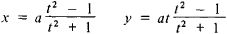traces a strophoid (see Figure 1, e) and traverses the location x = 0, y = 0 twice, namely, when t = − 1 and t = +1.

(3) In analytic geometry we also define a plane curve by an equation

F(x,y)= 0

and a space curve by two equations

F(x, y, z) = 0 G(x, y, z) = 0

By a (plane) algebraic curve we mean a curve defined by an equation

F(x,y) = 0

where F(x, y) is an entire algebraic function, that is, a polynomial of some degree n ≥ 1. Two polynomials F1(x, y) and F2(x, y) are said to define the same algebraic curve if and only if there exists a constant c ≠ 0 such that the equation

F1(x,y) = cF2(x,y)

is satisfied identically.

Thus, all polynomials that define a given curve have the same degree n called the degree of the curve. For example, in analytic geometry it is commonly assumed that the equation

(x - y)2 = 0

defines a quadratic curve, namely, the line x – y = 0 taken twice.

It should be noted in connection with the last example, however, that it is often useful to limit oneself to considering irreducible algebraic curves, that is, curves for which the defining polynomial F does not admit a representation F = GH, where G and H are nonconstant polynomials. Subsequently, in section (4), only this case will be considered.

A point (x0, y0) of the curve F(x, y) = 0 is said to have multiplicity m if the expansion of F(x, y) in powers of ξ = xx0 and η = y — y0 begins with terms of degree m (the degree of a term is the sum of the degrees of ξ and η). In the case of m = 2, that is, in the case of a double point

F(x,y) = a11 (x - x0)2 + 2a12 (x - x0)(y - y0) + a22(y - y0)2 + ...

where the dots stand for terms of higher order. By using the discriminant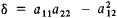it is possible to determine the nature of the double point.

(4) Often, especially in the study of algebraic curves, it is useful to assume the viewpoint of complex projective geometry, that is, to consider points at infinity and imaginary points in addition to points of the real Euclidean plane (or space). Only with this approach (and with due consideration for the multiplicity of each point of intersection) does, for example, the assertion that two curves of degrees n and m intersect in mn points become valid. For m = 1 this result can be used to define the degree of a curve as the number n of its points of intersection with a line.

From the projective point of view it is natural to define a plane curve by means of the homogeneous equation

F(x1, x2, x3) = 0

connecting the homogeneous coordinates x1, x2, x3 of its points. According to the principle of duality, the curve can also be defined by the equation

Φ(ξ1, ξ2, ξ3) = 0

which relates the homogeneous coordinates of its tangents. Thus, in addition to the degree of a curve (the degree of the equation F = 0), we are led to the concept of the class of a curve—the degree of the equation Φ = 0. The class of an algebraic curve may also be defined as the number of tangents that can be drawn to a curve from an arbitrary point.

(5) The refinements and generalizations of the concept of curve that were examined above (in sections 2–4) are linked in an essential manner to the appropriate algebraic and analytic apparatus. In contrast to this, modern topology has raised the problem of revising the concept of a curve as a set of points with a view to dispensing with algebraic or analytic descriptions of the set.

If we proceed from the parametric definition of a curve as a continuous function P = ϕ (t), where t varies over a segment atb, but consider only the resulting set of points while ignoring their order, then we obtain the concept of curve formulated in the 1890’s by C. Jordan. It turns out that any locally connected continuum, in particular a square, triangle, or cube, may be such a continuous image of a segment. Therefore, mathematicians now usually prefer to speak not of a curve in Jordan’s sense but of locally connected, or Jordan, continua. A one-to-one continuous image of a segment is called a simple, or Jordan, arc. A one-to-one continuous image of a circle is a simple closed curve. Simple arcs and simple closed curves, however, do not exhaust the point sets that may be called curves.

In modern topology we use a definition of curve, introduced in 1921 by P. S. Urysohn, which is neither excessively general nor excessively restrictive. Urysohn defined a curve as an arbitrary continuum of dimension one. A continuum has dimension one if for any ∊ Ⓗ 0 it can be represented as the union of a finite number of closed sets of diameter less than ∊, such that no three of them have a point in common. A plane continuum is a curve in Urysohn’s sense if and only if it contains no interior points. G. Cantor previously (in the 1870’s) used this property to characterize plane curves. Although Cantor’s definition is applicable only to plane curves, general curves in Urysohn’s sense are also sometimes called Cantor curves.

A. N. KOLMOGOROV

(6) Ancient mathematicians studied quadratic curves (the ellipse, hyperbola, and parabola). They also investigated a number of noteworthy algebraic curves of higher order as well as some transcendental (nonalgebraic) curves. The systematic study and classification of curves became possible with the development of analytic geometry (R. Descartes).

We now describe the best known cubic curves.

(a) Folium of Descartes (Figure 1, a). The equation in rectangular Cartesian coordinates is x3+ y3 – 3axy = 0. This curve was first defined in a letter from R. Descartes to P. Fermat in 1638. The complete form of the curve with an asymptote passing through the points (–a, 0) and (0, –a) was determined in 1692, by C. Huygens and Johann Bernoulli. The name “folium of Descartes” was introduced in the early 18th century.

(b) Witch of Agnesi (Figure 1, b). Consider a circle with diameter OC = a and a segment BDM such that OB : BD = OC : BM; the locus of points M is the witch of Agnesi (or versiera). Its equation in rectangular coordinates is y = a3/ (a2 + x2). The investigation of this curve is associated with the name of the Italian mathematician Maria Agnesi (1748).

(c) Cubical parabola (Figure 1, c). Its equation in rectangular coordinates is y = x3.

(d) Semicubical parabola (Figure 1, d), or Neile’s parabola. Its equation in Cartesian coordinates is y = cx3/2. The curve was named after the English mathematician W. Neile (1657), who found the length of its arc.

(e) Strophoid (Figure 1, e). Let AB be a fixed line and let C be a point at a distance CO = a ≠ 0 from AB. A line intersecting AB at the variable point N is rotated around C. If segments NM = NM′ = NO are laid off from N on both sides of AB, then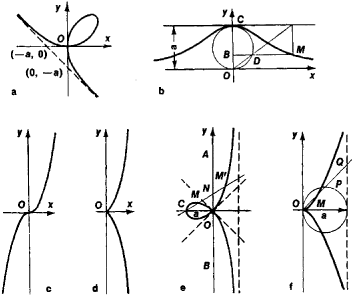Figure 1. Cubic algebraic curves: (a) folium of Descartes, (b) witch of Agnesi, (c) cubical parabola, (d) semicubical parabola, (e) strophoid, and (f) cissoid of Diocles

the locus of the points M and M′ for all positions of the rotating ray CN is a strophoid. Its equation in rectangular coordinates is y2 = x2 (a + x)/(a – x); in polar coordinates it is ρ = — a cos 2ϕ/cos ϕ. The strophoid was first investigated by E. Torricelli in 1645, and the name was introduced in the mid-19th century.

(f) Cissoid of Diocles (Figure 1, f), the locus of the points M for which OM = PQ (where P is an arbitrary point of the generating circle of diameter a). Its equation in rectangular coordinates is y2 = x3/ (a − x); in polar coordinates it is ρ = a sin2ϕ/cos ϕ. The ancient Greeks considered only the part of the cissoid in the interior of the generating circle. Together with the arc of the circle, this part forms a figure resembling a leaf of ivy (hence the name cissoid, meaning ivy). The existence of infinite branches was established independently in the 17th century by the French mathematician G. P. de Roberval and the Belgian mathematician R. F. de Sluse.

The best-known quartic and higher-degree curves are as follows.

(a) Cardioid (Figure 2, a), a curve described by a point M of a circle of radius a that rolls without sliding along a fixed circle of the same radius. Its equation in rectangular coordinates is (x2 + y2 – 2ax)2 = 4a(x2 + y2); in polar coordinates it is ρ = 2a(1 + cos ϕ).

(b) Conchoid of Nicomedes (Figure 2, b), a curve generated by increasing or decreasing the radius-vector of each point of a given line by the same quantity d; thus OM = OP − d or OM— OP + d. If the distance from the pole O to the given line is a, then the equation of the conchoid in rectangular coordinates is (xa)2 (x2 + y2) – d2x2 = 0, and in polar coordinates it is ρ = a/cos ϕ ± d. This curve was first examined by the ancient Greek geometer Nicomedes (c. 250–150 B.C.), who used it to solve the problems of the trisection of an angle and doubling the cube.

(c) Lemniscate of Bernoulli (Figure 2, c), a figure-eight curve; the locus of points, the product of whose distances from the foci F1(—a, 0) and F2(a, 0) is equal to a2. Its equation in rectangular coordinates is (x2 + y2)2 – 2a2(x2y2) = 0; in polar coordinates it is ρ2 = 2a2 cos 2ϕ. This curve was first examined by Jakob Bernoulli in 1694. The lemniscate is a particular case of the ovals of Cassini and sinusoidal spirals.

(d) Ovals of Descartes (Figure 2, d), the loci of points M whose distances from two fixed points F1 and F2, called the foci, multiplied by given numbers have a constant sum c, that is, mMF1 + nMF2 = c. The equation in rectangular coordinates is

(x2 + y2 – 2rx)2 - l2(x2 + y2) - k= 0

where r, l, and k are certain constants related to the parameters m, n, and d; in polar coordinates it is

(n2 - m2)ρ2 + 2ρ(mc - n2d cos ϕ) + n2d2 - c2 = 0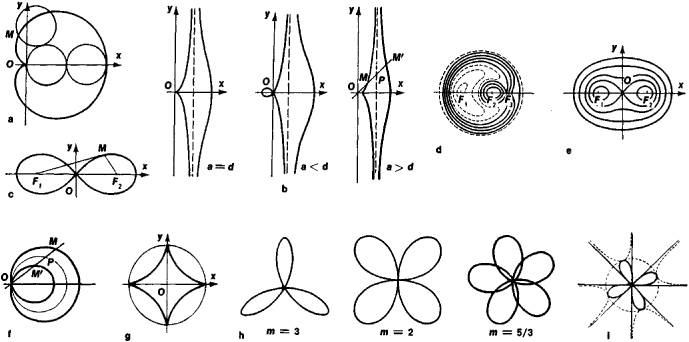Figure 2. Quartic and higher-degree algebraic curves: (a) cardioid, (b) conchoid of Nicomedes, (c) lemniscate of Bernoulli, (d) ovals of Descartes, (e) ovals of Cassini, (f) Pascal’s limaçon, (g) astroid, (h) roses, and (i) sinusoidal spiral

In addition to the foci F1 and F2, there is a third focus F3 that is equivalent to each of the first two. When m = 1 and n = 1, the oval of Descartes becomes an ellipse; when m = 1 and n = —1, it becomes a hyperbola. Pascal’s limaçon is also a particular case of an oval. Ovals were first studied by R. Descartes in 1637.

(e) Ovals of Cassini (Figure 2, e), the loci of points M, the product of whose distances from two given points is constant. Let F1 and F2 be points on the x-axis such that F1F2 = 2b and the product MF1 · MF2 = a2. Then the equation of the oval in rectangular coordinates is

(x2 + y2)2 - 2b2(a2 - y2) = a4 - b4

If a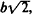then the oval of Cassini is a convex curve; if b < a <then the curve has the form of an oval with two bumps; when a = b, the oval of Cassini becomes a lemniscate; and, finally, when b > a, the oval of Cassini is a doubly connected curve. This curve was first examined by G. D. Cassini in the 17th century.

(f) Pascal’s limaçon (Figure 2, f), the locus of points M and M′ lying on the lines of a pencil (whose center O lies on a circle of radius R) at a distance a on either side of the point P of intersection of the lines of the pencil with the circle; thus, PM = PM′ = a. The equation of the resulting curve in rectangular coordinates is (x2 + y2 — 2Rx)2a2(x2 + y2) = 0; its equation in polar coordinates is ρ = 2R cos 0 + a. When a = 2R, the loop shrinks to a point, and Pascal’s limaçon becomes a cardioid. This curve was named after the French scientist Etienne Pascal (1588–1651), who first studied it.

(g) Astroid (Figure 2, g), a curve described by a point on a circle that rolls without sliding on the inside of a fixed circle whose radius is four times that of the moving circle. Its equation in rectangular coordinates is x2/3 + y2/3 = a2/3, where a is the radius of the fixed circle. The astroid is a sextic curve.

(h) Roses (Figure 2, h), curves whose equation in polar coordinates is ρ = a sin m ϕ; if m is a rational number, then the roses are algebraic curves of even degree. When m is odd, the rose consists of m leaves; when m is even, it consists of 2m leaves; and when m is rational, the leaves partially overlap.

(i) Sinusoidal spirals (Figure 2, i), curves whose equation in polar coordinates is ρm = am cos m ϕ; if m is a rational number, then these curves are algebraic. Particular cases are m = 1—a circle; m = — 1—a line; m = 2 —the lemniscate of Bernoulli; m = —2—an equilateral hyperbola; m = 1/2—a cardioid; and m = —1/2—a parabola. When m Ⓗ 0 is integral, the curve consists of m leaves, each of which lies within an angle π/m; when m Ⓗ 0 is rational, the leaves may partially overlap. If m < 0, then the curve consists of m infinite branches.

The transcendental curves are a large and interesting class. They include the graphs of trigonometric functions (such as the sine and tangent curves), the logarithmic function, the exponential function, hyperbolic functions, and the following curves.Figuie 3. Transcendental curves: (a) quadratrix, (b) tractrix, (c) catenary, (d) cycloid, (e) spiral of Archimedes, (f) hyperbolic spiral, (g) lituus, (h) logarithmic spiral, (i) Cornu spiral, and (j) si-ci spiral

(a) Quadratrix (Figure 3, a). Let the line MN uniformly rotate counterclockwise about the point O and the line A′B′ move uniformly from right to left, remaining parallel to OC. Further, while AB ′ moves from AB to OC, let MN rotate through a right angle and move from the position OA = r to the position OC. The locus of the points P of intersection of lines MN and AB′ is a quadratrix. Its equation in rectangular coordinates is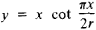Its equation in polar coordinates is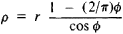The part of the quadratrix lying within the square OABC was known to ancient Greek mathematicians. The discovery of the quadratrix is ascribed to Hippias of Elis (fifth century B.C.), who used it to solve the problem of the trisection of an angle. Dino-stratus (fourth century B.C.) squared the circle using the quadratrix.

(b) Tractrix (Figure 3, b), a curve for which the length of a segment of the tangent from the point of tangency M to the point P of intersection with a given line is a constant a. Its equation in rectangular coordinates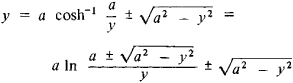(c) Catenary (Figure 3,c), the curve formed by a uniform flexible and inextensible cord suspended at its ends. Its equation in rectangular coordinates is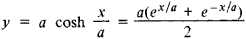(d) Cycloid (Figure 3, d), a curve described by a point P at a distance a from the center of a circle of radius r that rolls without sliding along a line. If P lies on the circumference of the circle (r = a), then the curve is an ordinary cycloid (Figure 3, d1); if P lies within the circle (r Ⓗ a), then the curve is a curtate cycloid (Figure 3, d2); and if the point lies outside the circle (r < a), then the curve is a prolate cycloid (Figure 3, d3). The last two curves are called trochoids. The parametric equations of a cycloid are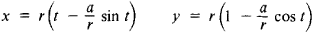Among the transcendental curves, spirals, which are plane curves going around a certain point an infinite number of times and approaching or receding from the point on each circuit, constitute a special class. If this point is chosen as the pole of a polar coordinate system, then the spiral is given by an equation ρ = f (ϕ) such that / (ϕ + 2π) > f (ϕ) or f (ϕ + 2π) < f (ϕ) for all ϕ. The best-known spirals include the following.

(e) Spiral of Archimedes (Figure 3, e), a curve described by a point moving uniformly along a line that rotates uniformly in the plane about the point O. Its equation in polar coordinates is ρ = a ϕ, where a is a constant. This spiral was studied by Archimedes (third century B.C.) in connection with the problems of the trisection of an angle and squaring the circle.

(f) Hyperbolic spiral (Figure 3, f), a curve described by a point M moving along a rotating line OA in such a way that its distance from the center of rotation varies inversely with the angle of rotation. Its equation in polar coordinates is ρ = a.

(g) Lituus (Figure 3, g), a curve whose equation in polar coordinates is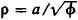. To each value of ϕ there correspond two values of ρ—one positive and one negative. The curve consists of two branches, each of which approaches the pole asymptotically.

(h) Logarithmic spiral (Figure 3, h), a curve whose equation in polar coordinates is ρ = ae. This curve was known to many 17th-century mathematicians.

(i) Cornu spiral (Figure 3, i), a curve consisting of two branches that are symmetric with respect to the origin. It has parametric equations.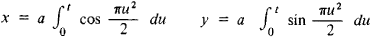This curve was used by the French physicist M. A. Cornu (1874) for the graphic solution of certain problems of diffraction of light.

(j) The si-ci spiral (Figure 3, j), a curve whose parametric equations are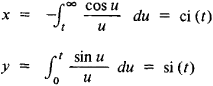where si (t) and ci (t) are the integral sine and integral cosine, respectively.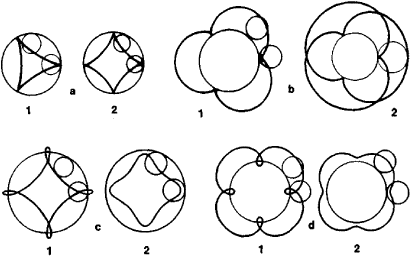Figure 4. Cycloidal curves: (a1) and (a2) hypocycloids, (b1) and (b2) epicycloids, (c1) prolate hypocycloid, (c2) curtate hypocycloid, (d1) prolate epicycloid, and (d2) curtate epicycloid

The cycloidal curves, which may be algebraic or transcendental, resemble the cycloid in the method of construction. These include the following curves.

(k) Hypocycloid (Figure 4, a1 and 4, a2), a curve described by a point on a circle that rolls without sliding along another circle within it. It has parametric equationswhere A is the radius of the fixed circle and a is the radius of the moving circle. The form of the curve depends on the ratio A/a.

(1) Epicycloid (Figure 4, b1 and 4, b2), a curve described by a point on a circle that rolls without sliding along another circle outside it. Its parametric equations are obtained from the equation of the hypocycloid by replacing a with —a.

(m) Prolate hypocycloid (epicycloid), a curve described by a point lying outside a circle that rolls without sliding along a circle in its interior or exterior (Figure 4, c1 and 4, d1). A curtate hypocycloid (epicycloid) is defined in a similar manner (Figure 4, c2 and 4, d2). Prolate and curtate hypocycloids and epicycloids are sometimes called hypotrochoids and epitrochoids.

V. I. BITIUTSKOV, IU. A. GOR’KOV, and A. B. IVANOV

### REFERENCES

Markushevich, A. I. Zamechatel’nye krivye, 2nd ed. Moscow-Leningrad, 1952.
Savelov, A. A. Ploskie krivye: Sistematika, svoistva, primeneniia (Spravochnoe rukovodstvo). Moscow, 1960.
Parkhomenko, A. S. Chto takoe liniia. Moscow, 1954.
Pogorelov, A. V. Differentsial’naia geometriia, 5th ed. Moscow, 1969.
Walker, R. Algebraicheskie krivye. Moscow, 1952. (Translated from English.)
Loria, G. Spezielle algebraische und transzendente ebene Kurven: Theorie und Geschichte, 2nd ed., vols. 1–2. Leipzig-Berlin, 1910–11.

## curve

[kərv]
(mathematics)
The continuous image of the unit interval.

## curve

1. Maths
a. a system of points whose coordinates satisfy a given equation; a locus of points
b. the graph of a function with one independent variable
2. a line representing data, esp statistical data, on a graph
3. short for French curve
Site: Follow: Share:
Open / Close